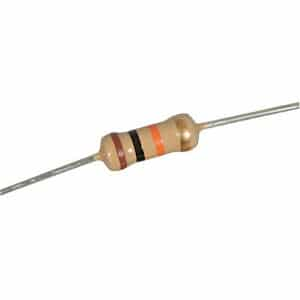# Easy Understanding 10k Ohm Resistor Color CodeA resistor is a fundamental passive component for an electrical circuit. It provides a specific resistance to the circuit. Now we will learn how to get a 10k ohm resistor color code.

Contents

## 10k Ohm Resistor Color Code

10k ohm resistor color code is shown with the color of 4 bands on it. If we want to get the 10k ohm, we should find a resistor with the color of:

 Band Color Band Color Value 1st Brown 1 2nd Black 0 3rd Orange 1000 4th Gold ±5%

Summary : (1) (0) (x1000) (+-5%) = 10k +-5% ohm

## 10k Ohm Resistor Illustration

Below is the illustration of a 10k ohm resistor. The size and the color may differ from one to another.

The color doesn’t have any meaning, but the size will determine the power rating. The bigger its size, the bigger its power rating.## 10k Ohm Resistor Calculation

For better understanding let us see the resistor color code chart below.## Real Picture of 10k Ohm Resistor

Below is the real picture of 10k ohm resistor:## Measurement of 10k Ohm Resistor

If we use a multimeter or ohmmeter we will get the resistance of 10k +-5%. The 5% is the tolerance from the gold band. It means the resistance will range from 9.5k to 10.5k ohm.1. How do I know if I have a 10k ohm resistor?A 10k ohm resistor has 4 color band: brown, black, orange, and gold for 5% tolerance, respectively.

2. What color is a 100 ohm resistor?

A 100 ohm resistor has 4 color band: brown, black, brown, and gold for 5% tolerance, respectively.

3. What does a 1k ohm resistor look like?

A 1k ohm resistor has 4 color band: brown, black, red, and gold for 5% tolerance, respectively.